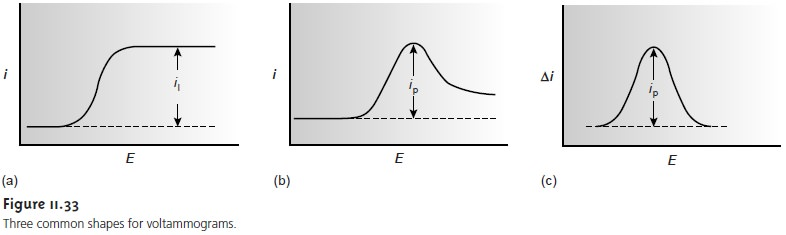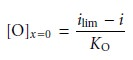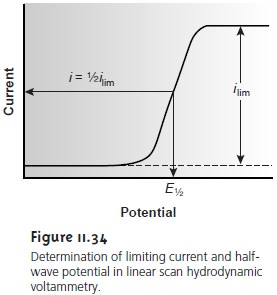Home | | Modern Analytical Chemistry | Quantitative and Qualitative Aspects of Voltammetry

# Quantitative and Qualitative Aspects of Voltammetry

In this section we consider how quantitative and qualitative information may be extracted from a voltammogram.

Quantitative and Qualitative Aspects of Voltammetry

Earlier we described a voltammogram as the electrochemical equivalent of a spec- trum in spectroscopy. In this section we consider how quantitative and qualitative information may be extracted from a voltammogram. Quantitative information is obtained by relating current to the concentration of analyte in the bulk solution. Qualitative information is obtained from the voltammogram by extracting the standard-state potential for the redox reaction. For simplicity we only consider voltammograms similar to that shown in Figure 11.33a.## Determining Concentration

Let’s assume that the redox reaction at the working electrode is

O+ ne– < = = = = > R    ……………11.34

and that initially only O is present in the bulk solution. The current is determined by the rate at which O diffuses through the fixed diffusion layer (see Figure 11.32), and is given by equation 11.33, or

i = KO([O]bulk [O]x=0)    ……………11.35

where KO is a constant equal to nFADO/ δ. When the limiting current is reached, the concentration of O at the electrode surface is zero, and this equation simplifies to

ilim = KO[O]bulk    ……………11.36

Thus, the limiting current, ilim, is a linear function of the concentration of O in bulk solution, and a quantitative analysis is possible using any of the standardization methods. Equations similar to equation 11.35 can be devel- oped for other forms of voltammetry, in which peak currents are related to the ana- lyte’s concentration in bulk solution.## Determining the Standard-State Potential

To extract the standard-state potential, or formal potential, for reaction 11.34 from a voltammogram, it is necessary to rewrite the Nernst equation11.37

in terms of current instead of the concentration of O and R. Substituting equation 11.36 into equation 11.35 and rearranging gives11.38

To derive a similar equation for the concentration of R at the electrode surface we note thatSince the concentration of R in bulk solution is zero, this equation simplifies to

i = KR[R]x=011.38where E1/2 is the half-wave potential (Figure 11.34). If KO is approximately equal to KR, which is often the case, then the half-wave potential is equal to the standard-state potential. Note that equation 11.41 is only valid if the redox reaction is electrochemically reversible. Voltammetric techniques giv- ing peak potentials also can be used to determine a redox reaction’s standard- state potential.Study Material, Lecturing Notes, Assignment, Reference, Wiki description explanation, brief detail
Modern Analytical Chemistry: Electrochemical Methods of Analysis : Quantitative and Qualitative Aspects of Voltammetry |